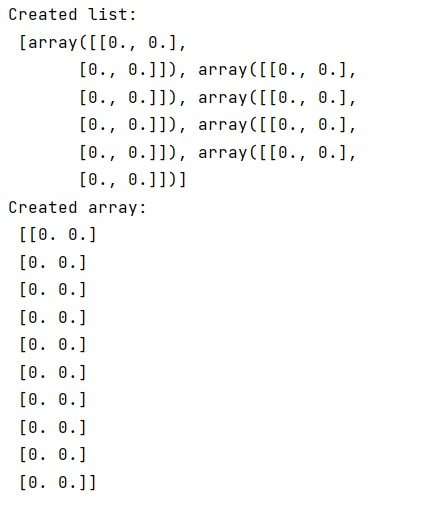# How to convert list of numpy arrays into single numpy array?

Learn, how to convert list of numpy arrays into single numpy array in Python?
Submitted by Pranit Sharma, on February 14, 2023

NumPy is an abbreviated form of Numerical Python. It is used for different types of scientific operations in python. Numpy is a vast library in python which is used for almost every kind of scientific or mathematical operation. It is itself an array which is a collection of various methods and functions for processing the arrays.

## Converting list of numpy arrays into single numpy array

Suppose that we are given a list of 2-dimensional numpy arrays and we need to convert this list into a single 2-dimensional numpy array.

Hence, we need to stack each of the numpy array one below another. For this purpose, we will use numpy.vstack() method.

The vstack() stacks arrays in sequence vertically (row-wise). This function makes the most sense for arrays with up to 3 dimensions.

For instance, for pixel-data with a height (first axis), width (second axis), and r/g/b channels (third axis). The functions concatenate, stack and block provide more general stacking and concatenation operations.

Let us understand with the help of an example,

## Python code to convert list of numpy arrays into single numpy array

```# Import numpy
import numpy as np

# Creating a list of np arrays
l = []

for i in range(5):
l.append(np.zeros([2,2]))

# Display created list
print("Created list:\n",l)

# Creating a ndarray from this list
arr = np.vstack(l)

# Display created array
print("Created array:\n",arr)
```

Output: